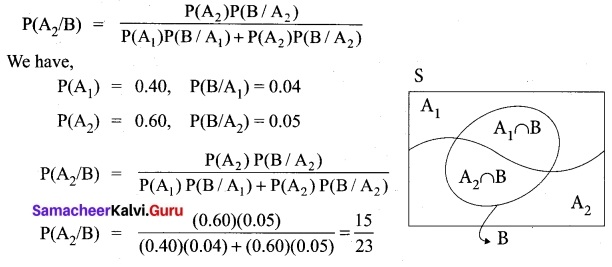Students can Download Tamil Nadu 11th Maths Model Question Paper 2 English Medium Pdf, Tamil Nadu 11th Maths Model Question Papers helps you to revise the complete Tamilnadu State Board New Syllabus and score more marks in your examinations.

## TN State Board 11th Maths Model Question Paper 2 English Medium

General Instructions:

1. The question paper comprises of four parts.
2. You are to attempt all the parts. An internal choice of questions is provided wherever applicable.
3. All questions of Part I, II, III and IV are to be attempted separately.
4. Question numbers 1 to 20 in Part I are Multiple Choice Questions of one mark each.
These are to be answered by choosing the most suitable answer from the given four alternatives and writing the option code and the corresponding answer
5. Question numbers 21 to 30 in Part II are two-mark questions. These are to be answered in about one or two sentences.
6. Question numbers 31 to 40 in Part III are three-mark questions. These are to be answered in above three to five short sentences.
7. Question numbers 41 to 47 in Part IV are five-mark questions. These are to be answered in detail Draw diagrams wherever necessary.

Time: 2.30 Hours
Maximum Marks: 90

PART – I

Choose the correct answer. Answer all the questions. [20 × 1 = 20]

Question 1.
The range of the function $$\frac{1}{1-2sin x}$$ is………..
(a) (-∞, -1) ∪($$\frac{1}{3}$$, ∞)
(b) (-1, -1)
(c) (-1, $$\frac{1}{3}$$)
(d) (-∞, -1] ∪[$$\frac{1}{3}$$, ∞)
Solution:
(d) (-∞, -1] ∪[$$\frac{1}{3}$$, ∞)

Question 2.
The value of log√2 512 is……….
(a) 16
(b) 18
(c) 9
(d) 12
Solution:
(b) 18Question 3.
If a and b are the roots of the equation x² – kx + 16 = 0 and satisfy a² + b² = 32 then the value of k is………..
(a) 10
(b) -8
(c) -8, 8
(d) 6
Solution:
(c) -8, 8

Question 4.
The value of log9 27 is …………
(a) $$\frac{2}{3}$$
(b) $$\frac{3}{2}$$
(c) $$\frac{3}{4}$$
(d) $$\frac{4}{3}$$
Solution:
(b) $$\frac{3}{2}$$

Question 5.
The value of $$\frac{\sin 3 \theta+\sin 5 \theta+\sin 7 \theta+\sin 9 \theta}{\cos 3 \theta+\cos 5 \theta+\cos 7 \theta+\cos 9 \theta}$$ = ………….
(a) tan 3θ
(b) tan 6θ
(c) cot 3θ
(d) cot 6θ
Solution:
(b) tan 6θ

Question 6.
In 3 fingers the number of ways 4 rings can be worn in ways.
(a) 4³ – 1
(b) 34
(c) 68
(d) 64
Solution:
(d) 64

Question 7.
Everybody in a room shakes hands with everybody else. The total number of shake hands is 66. The number of persons in the room is……….
(a) 11
(b) 12
(c) 10
(d) 6
Solution:
(b) 12

Question 8.
The H.M of two positive number whose AM and G.M. are 16, 8 respectively is
(a) 10
(b) 6
(c) 5
(d) 4
Solution:
(d) 4

Question 9.
The co-efficient of the term independent of x in the expansion of (2x + $$\frac{1}{3x}$$)6 is……….
(a) $$\frac{160}{27}$$
(b) $$\frac{160}{37}$$
(c) $$\frac{80}{3}$$
(d) $$\frac{80}{9}$$
Solution:
(a) $$\frac{160}{27}$$

Question 10.
The value of $$\left|\begin{array}{lll} a & 0 & 0 \\ 0 & b & 0 \\ 0 & 0 & c \end{array}\right|^{2}$$ is……….
(a) abc
(b) -abc
(c) 0
(d) a²b²c²
Solution:
(d) a²b²c²Question 11.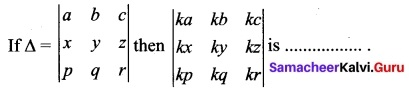(a) Δ
(b) kΔ
(c) 3kΔ
(d) k³Δ
Solution:
(d) k³Δ

Question 12.
A vector makes equal angle with the positive direction of the co-ordinate axes then each angle is equal to………..
(a) cos-1 ($$\frac{1}{3}$$)
(b) cos-1 ($$\frac{2}{3}$$)
(c) cos-1 ($$\frac{1}{√3}$$)
(d) cos-1 ($$\frac{2}{√3}$$)
Solution:
(c) cos-1 ($$\frac{1}{√3}$$)

Question 13.
If the centroids of AABC and A’B’C’ are respectively G and G’ then $$\bar{AA’}$$ + $$\bar{BB’}$$ + $$\bar{CC’}$$…………
(a) $$\bar{GG’}$$
(b) 3$$\bar{GG’}$$
(c) 2$$\bar{GG’}$$
(d) 0
Solution:
(b) 3$$\bar{GG’}$$

Question 14.
If f(x) = \left\{\begin{aligned} k x^{2} & \text { for } \quad x \leq 2 \\ 3 & \text { for } \quad x>2 \end{aligned}\right. is continuous at x = 2 then the value of k is ………..
(a) $$\frac{3}{4}$$
(b) 0
(c) 1
(d) $$\frac{4}{3}$$
Solution:
(d) $$\frac{4}{3}$$

Question 15.
If x = $$\frac{1-t²}{1+t²}$$ and y = $$\frac{2t}{1+t²}$$ then $$\frac{dx}{dy}$$ = ……….
(a) $$\frac{y}{x}$$
(b) $$\frac{-y}{x}$$
(c) –$$\frac{x}{y}$$
(d) $$\frac{x}{y}$$
Solution:
(c) –$$\frac{x}{y}$$

Question 16.
$$\lim _{x \rightarrow \infty}\left(\frac{x^{2}+5 x+3}{x^{2}+x+3}\right)^{x}$$ is ……….
(a) e4
(b) e²
(c) e³
(d) 1
Solution:
(a) e4

Question 17.
$$\int \frac{e^{x}\left(x^{2} \tan ^{-1} x+\tan ^{-1} x+1\right)}{x^{2}+1}$$ dx is ……….
(a) extan-1(x + 1) + c
(b) tan-1(ex) + c
(c) ex $$\frac{tan^{-1}x}{2}$$ + c
(d) extan-1x + c
Solution:
(d) extan-1x + c

Question 18.
$$\int \frac{\sec x}{\sqrt{\cos 2 x}}$$ dx = ………
(a) tan-1(sin x) + c
(b) 2 sin-1(tan x) + c
(c) tan-1(cos x) + c
(d) sin-1(tan x) + c
Solution:
(d) sin-1(tan x) + cQuestion 19.
$$\int \frac{e^{6 \log x}-e^{5 \log x}}{e^{4 \log x}-e^{3 \log x}}$$
(a) x + c
(b) $$\frac{x³}{3}$$ + c
(c) $$\frac{3}{x³}$$ + c
(d) $$\frac{1}{x²}$$ + c
Solution:
(b) $$\frac{x³}{3}$$ + c

Question 20.
It is given that the events A and B are such that P(A) = $$\frac{1}{4}$$, P(A/B) = $$\frac{1}{2}$$ and P(B/A) = $$\frac{2}{3}$$ then P(B) = …………
(a) $$\frac{1}{6}$$
(b) $$\frac{1}{3}$$
(c) $$\frac{2}{3}$$
(d) $$\frac{1}{2}$$
Solution:
(b) $$\frac{1}{3}$$

PART- II

II. Answer any seven questions. Question No. 30 is compulsory.

Question 21.
Find x such that -π ≤ x ≤ π and cos 2x = sin x
Solution:
We have cos 2x = sin x which gives
2sin² x + sin x – 1 = 0
The roots of the equation are sin x = $$\frac{-1±3}{4}$$ = -1 (or) $$\frac{1}{2}$$
Now, sin x = $$\frac{1}{2}$$ ⇒ x = $$\frac{π}{6}$$, $$\frac{5π}{6}$$
Also sin x = -1 ⇒ x = $$\frac{π}{2}$$
Thus x = $$\frac{π}{2}$$, $$\frac{π}{6}$$, $$\frac{5π}{6}$$

Question 22.
If (n-1)P3 : nP4 = 1 : 10 find n.
Solution: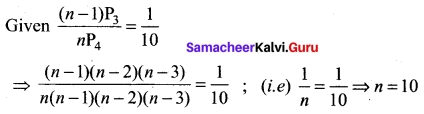Question 23.
Find the 18th and 25th terms of the sequence defined by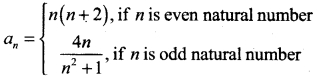Solution:
When n = 18 (even)
an = n(n + 2) = 18(18 + 2) = 18 (20) = 360
When n = 25 (odd)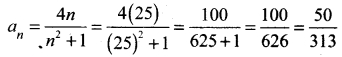Question 24.
Show that the lines are 3x + 2y + 9 = 0 and 12x + 8y – 15 = 0 are parallel lines.
Solution: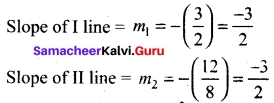Here m1 = m2 ⇒ the two lines are parallel.

Question 25.
Prove that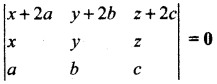Solution: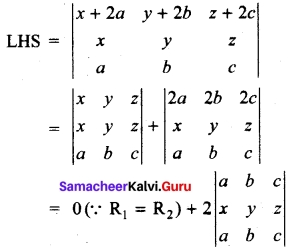= 0 + 2(0) (∴ R1 = R3)
= 0 = RHS

Question 26.
Find the value of λ for which the vectors $$\vec{a}$$ = 2$$\vec{i}$$ + λ$$\vec{j}$$ + $$\vec{k}$$ and $$\vec{b}$$ = $$\vec{i}$$ – 2$$\vec{j}$$ + 3$$\vec{k}$$ are perpendicular.
Solution:
When $$\vec{a}$$ and $$\vec{b}$$ are ⊥r If then $$\vec{a}$$.$$\vec{b}$$ = 0
$$\vec{a}$$ ⊥r $$\vec{b}$$ ⇒ $$\vec{a}$$.$$\vec{b}$$ = 0
(2) (1) + (λ) (-2) + (1) (3) = 0 ⇒ λ = 5/2Question 27.
If y = $$\frac{tan x}{x}$$ and $$\frac{dx}{dy}$$
Solution:
now y = $$\frac{u}{v}$$ ⇒ y’ =$$\frac{vu’-uv’}{v²}$$
u = tan x ⇒ u’ = sec²x
v = x ⇒ v’ = 1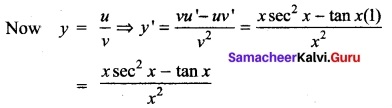Question 28.
Evaluate $$\int \sqrt{25 x^{2}-9}$$ dx
Solution: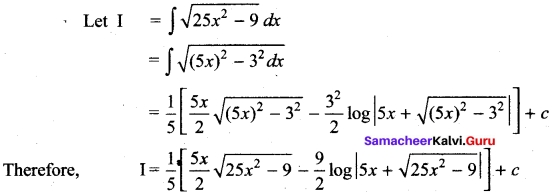Question 29.
If A and B are two independent events such that
P(A) = 0.4 and P(A ∪ B) = 0.9. Find P(B).
Solution:
P(A ∪ B) = P(A) + P(B) – P(A ∩ B)
P(A ∪ B) = P(A) + P(B) – P(A).P(B)(since A and B are independent)
That is, 0.9 = 0.4 + P(B) – (0.4) P(B)
0.9 – 0.4 = (1 – 0.4) P(B)
Therefore, P(B) = $$\frac{5}{6}$$

Question 30.
A rope of length 12 m is given. Find the largest area of the triangle formed by this rope and find the dimensions of the triangle so formed.
Solution:
The largest triangle will be an equilateral triangle
∴ side of the triangle = $$\frac{12}{3}$$ = 4 m = a
Area of the triangle = $$\frac{a²√3}{4}$$ = $$\frac{4²√3}{4}$$ = 4√3 sq.m

PART – III

III. Answer any seven questions. Question No. 40 is compulsory. [7 × 3 = 21]

Question 31.
Let A = {a, b, c} and R = {(a, a), (b, b), (a, c)}. Write down the minimum number of ordered pairs to be included to R to make it

1. reflexive
2. symmetric
3. transitive
4. equivalence

Solution:

1. (c, c)
2. (c, a)
3. nothing
4. (c, c) and (c, a)

Question 32.
Solve 2|x + 1| – 6 ≤ 7 and graph the solution set in a number line.
Solution:
2|x + 1| – 6 ≤ 7
⇒ 2|x + 1| ≤ 7 + 6 (= 13)
⇒ |x + 1| ≤ $$\frac{13}{2}$$
⇒ x + 1 > $$\frac{-13}{2}$$
x + 1 > $$\frac{-13}{2}$$
⇒ x > $$\frac{-13}{2}$$ -1 (= $$\frac{-15}{2}$$) ……….(1)
(or) x + 1 > $$\frac{13}{2}$$
x + 1 < $$\frac{-13}{2}$$
⇒ x < $$\frac{13}{2}$$ -1 (= $$\frac{11}{2}$$) ……….(2)
From (1) and (2) $$\frac{-15}{2}$$ ≤ x ≤ $$\frac{11}{2}$$

Question 33.
If the different permutations of all letters of the word BHASKARA are listed as in a dictionary, how many strings are there in this list before the first word starting with B?
Solution:
The required number of strings is the total number of strings starting with A and using the letters A, A, B, H, K, R, S = $$\frac{7!}{2}$$ = 2520

Question 34.
Find the sum up to n terms of the series: 1 + $$\frac{6}{7}$$ + $$\frac{11}{49}$$ + $$\frac{16}{343}$$ + ….
Solution:
Here a = 1, d = 5 and r = $$\frac{1}{7}$$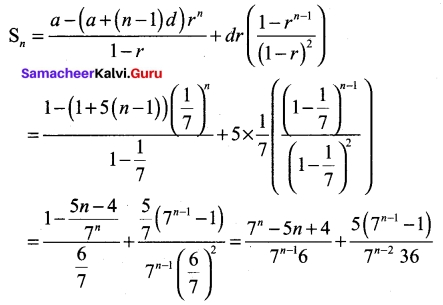Question 35.
Area of the triangle formed by a line with the coordinate axes, is 36 square units. Find the equation of the line if the perpendicular drawn from the origin to the line makes an angle of 45° with positive the x-axis.
Solution:
Let p be the length of the perpendicular drawn from the origin to the required line.
The perpendicular makes 45° with the x-axis.
The equation of the required line is of the form,
x cos α + y sin α = p
⇒ x cos 45° + y sin 45° = p
⇒ x + y= √2 P
This equation cuts the coordinate axes at A(√2p, 0) and B (o, √2p).
Area of the ΔOAB is $$\frac{1}{2}$$ × √2p × √2p = 36 ⇒ p = 6 (∵ p is positive)
Therefore the equation of the required line is x + y = 6 √2

Question 36.
If AT = $$\left(\begin{array}{cc} 4 & 5 \\ -1 & 0 \\ 2 & 3 \end{array}\right)$$ and B = $$\left(\begin{array}{ccc} 2 & -1 & 1 \\ 7 & 5 & -2 \end{array}\right)$$ verify that (A – B)T = AT – BT
Solution:
(A – B)T = AT – BT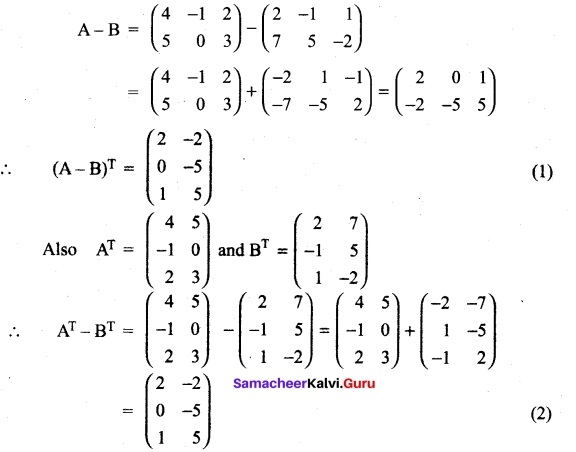Here (1) and (2) ⇒ (A – B)T = AT – BT

Question 37.
For any vector $$\vec{a}$$ prove that $$|\vec{a} \times \hat{i}|^{2}+|\vec{a} \times \hat{j}|^{2}+|\vec{a} \times \hat{k}|^{2}=2|\vec{a}|^{2}$$
Solution:
Let = $$\vec{a}$$ = a1$$\hat{i}$$ + a2$$\hat{j}$$ + a3$$\hat{k}$$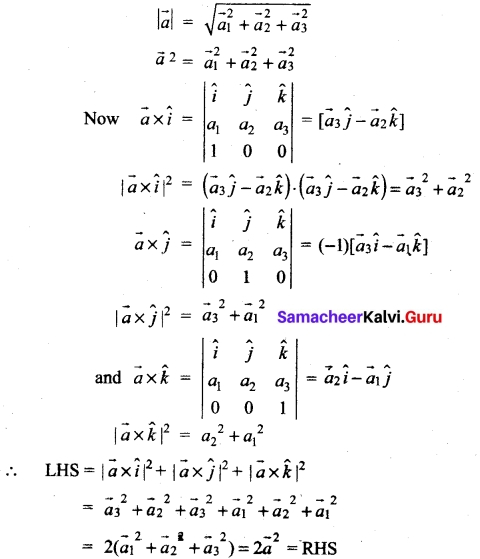Question 38.
Given y = cos-1 $$\left(\frac{1-x^{2}}{1+x^{2}}\right)$$ find $$\frac{dx}{dy}$$
Solution:
put x = tan θ
so $$\left(\frac{1-x^{2}}{1+x^{2}}\right)$$ = $$\left(\frac{1-tan^{2}θ}{1+tan{2}θ}\right)$$ = 2 cos θ
y = cos-1 (cos 2θ) = 2θ
⇒ $$\frac{dy}{dθ}$$
Now x = tan θ
⇒ $$\frac{dx}{dθ}$$ = sec²θ
= 1 + tan² θ
= 1 + x²
so $$\frac{dx}{dy}$$ = $$\frac{dy}{dθ}$$/$$\frac{dx}{dθ}$$ = $$\frac{2}{1+x²}$$

Question 39.
A wound is healing in such a way that t days since Sunday the area of the wound has been decreasing at a rate of –$$\frac{3}{(t+2)²}$$ cm² per day. If on Monday the area of the wound was 2 cm²
(i) What was the area of the wound on Sunday?
(ii) What is the anticipated area of the wound on Thursday if it continues to heal at the same rate ?
Solution:
Let A be the area of wound at time ‘ t ‘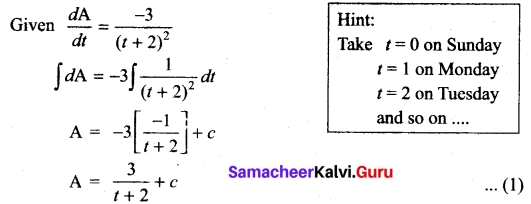By the given, condition area of the wound on monday is 2 cm²
⇒ A = 2, t = 1
⇒ 2 = $$\frac{3}{1+2}$$ + c
c = 1
∴ Area of wound at any day.
⇒ 1 ⇒ A = $$\frac{3}{1+2}$$ + 1
(i) The area of the wound on Sunday
t = 0 ⇒ A = $$\frac{3}{2}$$ + 1 = $$\frac{5}{2}$$ = 2.5 cm²
(ii) The area of the wound on Thursday
t = 4 ⇒ A = $$\frac{3}{6}$$ + 1 = $$\frac{1}{2}$$ + 1 = 1.5 cm²Question 40.
An integer is chosen at random from the first fifty positive integers. What is probability that the integer chosen is a prime or multiple of 4?
Solution:
S = {1, 2, 3, ……. ,50} ∴ n(S) = 50
Let A be the event of getting prime number.
∴ A = {2, 3, 5, 7, 11, 13, 17, 19, 23, 29, 31, 37, 41, 43, 47}
n(A) = 15, so P(A) = 15/50
Let B be the event of getting number multiple of 4
∴B = {4, 8, 12, 16, 20, 24, 28, 32, 36, 40, 44, 48}
n(B) = 12, so P(B) = 12/50
Here A and B are mutually exclusive. (i.e.,) A ∩ B = Φ
∴ P(A ∪ B) = P(A) + P(B) = 15/50+ 12/50 = 27/50

PART – IV

IV. Answer all the questions. [7 × 5 = 35]

Question 41 (a).
The formula for converting from Fahrenheit to Celsius temperatures is y = $$\frac{5x}{9}$$ – $$\frac{160}{9}$$ Find the inverse of this function and determine whether the inverse is also a function.
Solution: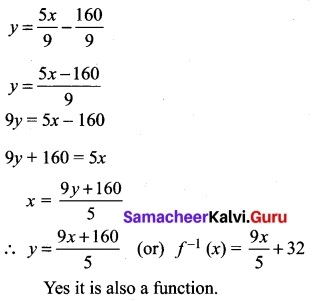[OR]

(b) If f : R → R is defined by f(x) = 2x – 3 prove that f is a injection and find its inverse.
Solution:
Method 1:
One-to-one: Let f(x) = f(y). Then 2x – 3 = 2y – 3; this implies that x = y. That is, f(x) = f(y) implies that x = y. Thus f is one-to-one.

Onto: Let y ∈ R. Let x =$$\frac{y+3}{2}$$ Then f(x) = 2($$\frac{y+3}{2}$$) -3 = y. Thus f is onto. This also can be proved by saying the following statement. The range of f is R (how?) which is equal to the co-domain and hence f is onto.

Inverse: Inverse: Let y = 2x – 3. Then y + 3 = 2x and hence x = $$\frac{y+3}{2}$$ Thus f-1 (y) = $$\frac{y+3}{2}$$. By replacing y as x, we get f-1 (x) = $$\frac{y+3}{2}$$

Method 2:
Let y = 2x – 3 then x = $$\frac{y+3}{2}$$. Let, g(y) = $$\frac{y+3}{2}$$
Now (g o f) (x) = g(f(x)) = g(2x – 3) = $$\frac{(2x-3)+3}{2}$$ = x
(f o g)(y) = f(g(y)) = f($$\frac{y+3}{2}$$) = 2($$\frac{y+3}{2}$$) -3 = y
This implies that f and g are bijections and inverses to each other. Hence f is a bijection and f-1(y) = $$\frac{y+3}{2}$$. Replacing y by x we get, f-1(x) = $$\frac{x+3}{2}$$

Question 42 (a).
If the equations x² – ax + b = 0 and x² – ex + f = 0 have one root in common and if the second equation has equal roots then prove that ae = 2(b + f).
Solution:
Let a be the common root
then a² – aα + b = 0 . . . .(1)
we are given that
x² – ex + f = 0 has equal roots.
So the roots will be α, β
Now sum of roots = 2α
= -(-e) ⇒ a = e/2
product of the roots
α × α = α²f
substituting a and α², values in (1) we get
f – a ($$\frac{e}{2}$$) + b = 0
f – $$\frac{ae}{2}$$ + b = 0
$$\frac{ae}{2}$$ = b + f ⇒ ae = 2(b + f)[OR]

(b) Prove that
cos θ + cos($$\frac{2π}{2}$$– θ) + (cos$$\frac{2π}{3}$$ + θ) = 0
Solution:
we have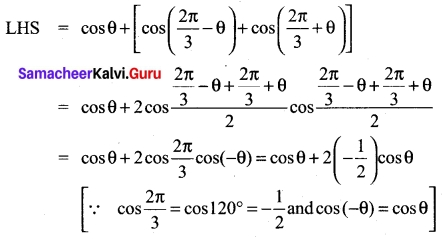cos θ – cos θ = 0 = RHS

Question 43 (a).
Find the number of strings that can be made using all letters of the word THING. If these words are written as in a dictionary, what will be the 85th string?
Solution:
(i) Number of words formed = 5! = 120
(ii) The given word is THING
Taking the letters in alphabetical order G H I N T
To find the 85th word
The No. of words starting with G = 4! = 24
The No. of words starting with H = 4! = 24
The No. of words starting with 1 = 4! = 24
The No. of words starting with NG = 3! = 6
The No. of words starting with NH = 3! = 6
The No. of words starting with NIGH = 1! = 1
Total = 85
So the 85th word is NIGHT

[OR]

(b) A straight line passes through a fixed point (6, 8). Find the locus of the foot of the perpendicular drawn to it from the origin O.
Solution:
Let the point (x1, y1) be (6, 8). and P (h, k) be a point on thr lequired locus.
Family of equations of the straight lines passing through the fixed point (x1, y1) is y – y1 = m (x – x1) ⇒ y – 8 = m(x – 6)
Since OP is perpendicular to the line (6.25)
m × ($$\frac{k-0}{h-0}$$) = -1 ⇒ m = –$$\frac{h}{k}$$
Also P(h, k) lies on (6.25)
⇒ k – 8 = – $$\frac{h}{k}$$(h – 6) ⇒ k (k – 8) = -h(h – 6) ⇒ h² + k² – 6h – 8k = 0
Locus of P (h, k) is x² + y² – 6x – 8y = 0

Question 44 (a).
If p is a real number and if the middle term in the expansion of ($$\frac{π}{2}$$ + 2)8 is 1120, find p.
Solution:
In the equation of ($$\frac{π}{2}$$ + 2)8, number of terms = 8 + 1 = 9 (odd)
∴ There is only one middle term i.e. ($$\frac{9+1}{2}$$)th or 5th term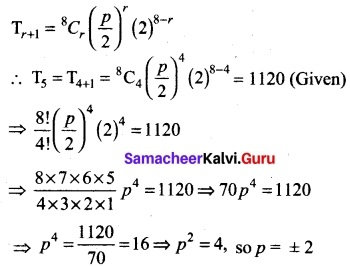[OR]

(b) Express the matrix A = $$\left[\begin{array}{rrr} 1 & 3 & 5 \\ -6 & 8 & 3 \\ -4 & 6 & 5 \end{array}\right]$$ the sum Of skew-symmetric matrices.
Solution: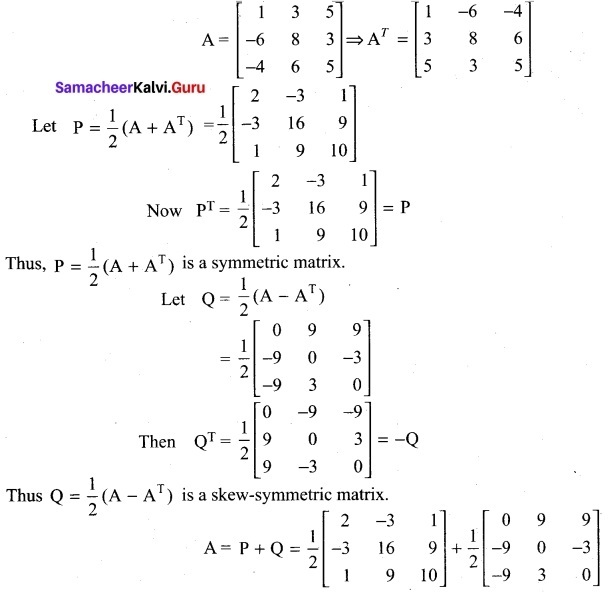Thus A is expressed as the sum of symmetric and skew-symmetric matrices.

Question 45 (a).
For any vector a prove that $$|\vec{a} \times \hat{i}|^{2}+|\vec{a} \times \hat{j}|^{2}+|\vec{a} \times \hat{k}|^{2}=2|\vec{a}|^{2}$$
Solution: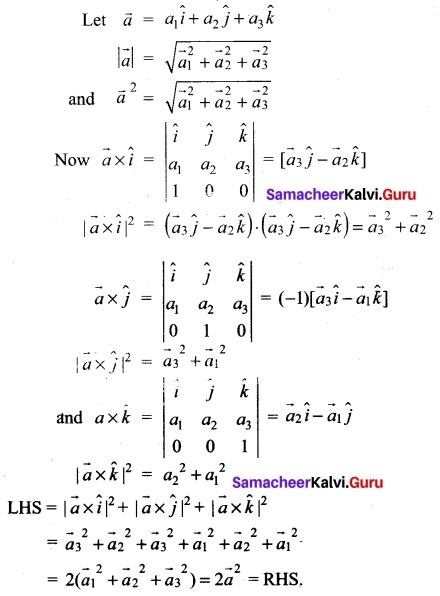[OR]

(b) If y = etan-1x show that (1 + x²) y” + (2x – 1) y’ = 0
Solution:
y = etan-1x
y’ = etan-1x ($$\frac{1}{1+x²}$$)
y’ = $$\frac{y}{1+x²}$$ ⇒ y’ (1 + x²) = y
differentiating w.r.to x
y’ (2x) + (1 + x²)(y”) = y’
(i.e.) (1 + x²) y” + y’(2x) – y’ = 0
(i.e.) (1 + x²)y” + (2x – 1) y’ = 0

Question 46 (a).
Evaluate $$\lim _{x \rightarrow \infty} \frac{(x+1)^{10}+(x+2)^{10}+\ldots+(x+100)^{10}}{x^{10}+10^{10}}$$
Solution: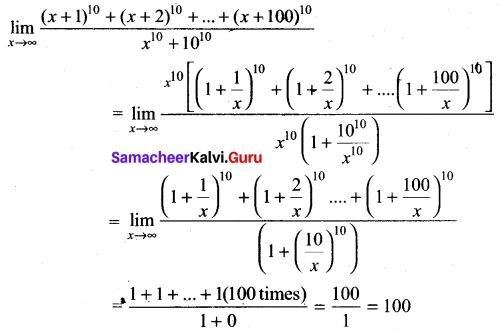[OR]

(b) Evaluate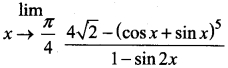Solution: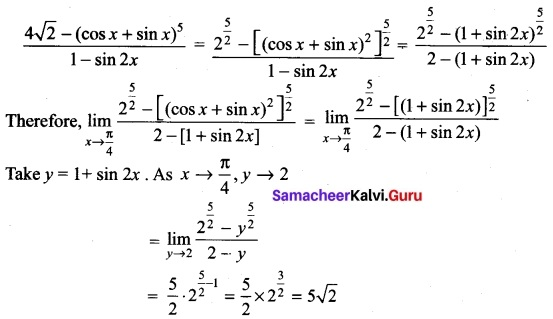Question 47 (a).
Evaluate $$\int \sin ^{-1}$$x dx
Solution:
Let 1 = sin-1x dx
u = sin-1x dv = dx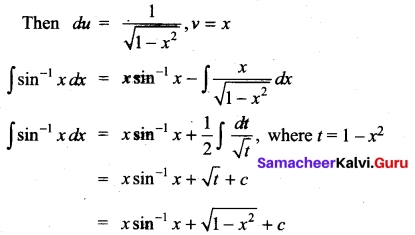[OR]

(b) A factory has two machines I and II. Machine I produces 40% of items of the output and Machine II produces 60% of the items. Further 4% of items produced by Machine I are defective and 5% produced by Machine II are defective. An item is drawn at random. If the drawn item is defective, find the probability that it was produced by Machine II.
Solution:
Let A1 be the event that the items are produced by Machine-I, A2 be the event that items are produced by Machine-II, Let B be the event of drawing a defective item. Now we are asked to find the conditional probability P(A2/B). Since A1, A2 are mutually exclusive and exhaustive events, by Bayes theorem,